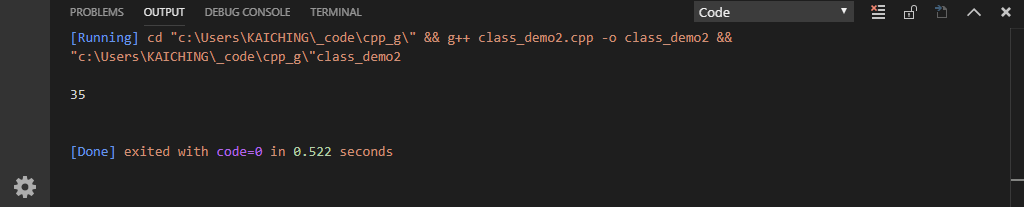# 單元 11 - 物件導向與封裝

``````class Demo {
// 宣告 public 的成員
public:
void set_a(int n);
void set_b(int n);
int get_a();
int get_b();
int DoSomething();

// 宣告 private 的成員
private:
int a;
int b;
};``````

set_a()set_b()修改函數 (mutator) ，就是俗稱的 setter ，至於 get_a()get_b()存取函數 (accessor) ，也就是是俗稱的 getter

``````// setter 與 getter 成員函數
void Demo::set_a(int n) {
a = n;
}

void Demo::set_b(int n) {
b = n;
}

int Demo::get_a() {
return a;
}

int Demo::get_b() {
return b;
}``````

``````#include <iostream>

using namespace std;

class Demo {
// 宣告 public 的成員
public:
void set_a(int n);
void set_b(int n);
int get_a();
int get_b();
int DoSomething();

// 宣告 private 的成員
private:
int a;
int b;
};

int Demo::DoSomething() {
// 改成呼叫 getter 成員函數
return get_a() + get_b();
}

// setter 與 getter 成員函數
void Demo::set_a(int n) {
a = n;
}

void Demo::set_b(int n) {
b = n;
}

int Demo::get_a() {
return a;
}

int Demo::get_b() {
return b;
}

int main(void) {
Demo t;
// 由呼叫 setter 設定成員變數
t.set_a(12);
t.set_b(23);

cout << endl << t.DoSomething() << endl << endl;

return 0;
}

/* 《程式語言教學誌》的範例程式
http://kaiching.org/
檔名：class_demo2.cpp
功能：示範定義類別
作者：張凱慶 */``````1. 物件導向程式設計有封裝、繼承及多型等三大基本特性。
2. 繼承像是親屬的重直關係（父母子女），多型則像是親屬的平行關係（兄弟姊妹）。
3. 封裝連帶的觀念就是資訊隱藏，類別的成員變數只能在類別中處理，因此要把資料成員宣告在 private 存取標籤下。
4. 外界要存取 private 的資料成員要透過 public 的存取函數與修改函數。

1. 物件導向程式設計有哪三大基本特性？
2. 什麼是封裝？為什麼要做封裝？
3. 什麼是繼承？繼承機制有什麼優點？
4. 什麼是多型？可以用什麼方式比擬嗎？
5. 什麼是存取函數與修改函數？為什麼要有存取函數與修改函數？

1. 寫一個程式 exercise1101.cpp ，利用 exercise1005.cpp 設計的 IntegerDemo ，替 value 加入存取函數與修改函數。
2. 寫一個程式 exercise1102.cpp ，利用 exercise1006.cpp 計算階層值的類別，替 value 加入存取函數與修改函數。
3. 寫一個程式 exercise1103.cpp ，利用 exercise1007.cpp 計算費氏數列的類別，替 value 加入存取函數與修改函數。